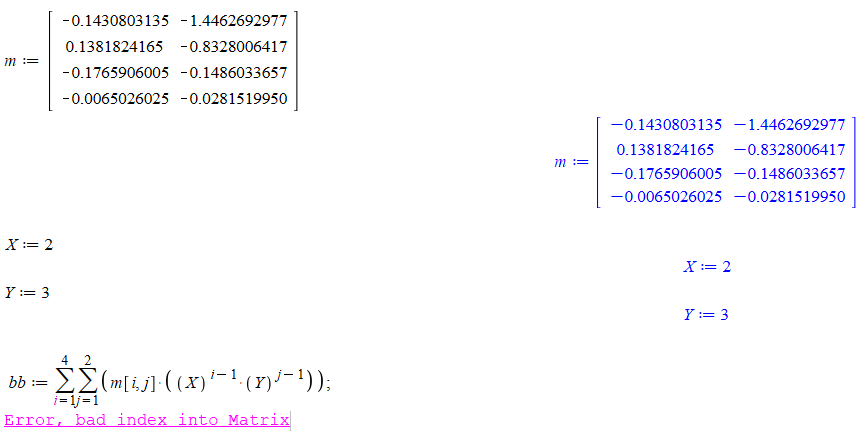## using arrows = curve gives error in DEplot. Any wo...

After I switched to using arrows = curve in the call to DEtools:-DEplot I found errors in some calls.

Is there a workaround other than not using this option? As phase plot looks like better with this option. For now, I will remove this option.

Here is an example

```restart;

ode:=diff(y(x),x)-1/(-x^2+1)^(1/2) = 0;
x_range:=-0.99 .. 0.99;
DEtools:-DEplot(ode,y(x),x =x_range,y = -1.6 .. 1.6,[y(0) = 0],arrows = 'curve')
```

Error, (in DEtools/DEplot/direction) cannot assign a complex value to a hardware float Array

But this works

```restart;

ode:=diff(y(x),x)-1/(-x^2+1)^(1/2) = 0;
x_range:=-0.99 .. 0.99;
DEtools:-DEplot(ode,y(x),x =x_range,y = -1.6 .. 1.6,[y(0) = 0])

```

I will report this to Maplesoft as it looks like a bug to me.

May be someone can find a workaround so I can use arrows = curve?

Maple 2023.2 on windows 10> restart;> ode:=diff(y(x),x)-1/(-x^2+1)^(1/2) = 0; x_range:=-0.99 .. 0.99; DEtools:-DEplot(ode,y(x),x =x_range,y = -1.6 .. 1.6,[y(0) = 0],arrows = 'curve')Error, (in DEtools/DEplot/direction) cannot assign a complex value to a hardware float Array

 > restart;> ode:=diff(y(x),x)-1/(-x^2+1)^(1/2) = 0; x_range:=-0.99 .. 0.99; DEtools:-DEplot(ode,y(x),x =x_range,y = -1.6 .. 1.6,[y(0) = 0])>

## Can Maple dsolve formally this ode?...

I recently answered a question concerning the Lane-Emden equation (see here LaneEmden) where the main topic was about finding its numerical solution.

The generic form of the Lane-Emden equation with parameter n is

```LaneEmden := n -> (Diff(xi^2*(Diff(theta(xi), xi)), xi)) = -theta(xi)^n * xi^2

d   /  2 / d            \\             n   2
n -> ---- |xi  |---- theta(xi)|| = -theta(xi)  xi
dxi \    \ dxi          //
```

I have just realized that I missed a "small" point in the original question: the OP ( @shashi598 ) wrote
"[...] Maple never comes out of evaluating [the] analytical solution when n=5 [...] ".
The important point here is that this solution (at least for some initial conditions) is known and simple (in the sense it doen't involve any special function).

So I tried for a few hours to verify this claim, and ended wondering myself if it might not be right?

Could you please tell me (I guess @shashi598 would be interested too in your return) if the differential equation LaneEmden(5) can be solved formally?
TIA.

Emden_equation.mw

EDITED:
After a little research it seems that very specigic method are used to build the analytic solution of the LaneEmden(n) (n not equal to 0, 1 and 5): serie expansions, homotopy, Adomian decomposition for instance.
I wasn't capable to find how the solution for LaneEmden(5) have been got for the first time (iseems to be atthe end of the 19th century).

## Insert a Matrix in Sum...

Hello everyone,

Can someone help me how to use a Matrix in sum?

Thanks## how I can find the solution of differential equati...

how I can find the solution of differential equations with dsolve?

Thesis_(1).mw

 >>>(1)
 >(2)
 >(3)
 >(4)
 >>(5)
 >(6)
 >>>>## improving phaseplot for system of ode's...

Given a system of 2 first order ode's which are autonomous (i.e. the RHS does not explicitly depend on time), one can make phase plot in Maple using DEtools:-DEplot

I am trying to get my Maple output to look like I get with Mathematica. I am getting close, but there are two issues. First, here is what I get in Maple

```restart;

ode1:=diff(x(t),t)=2*x(t)*y(t);
ode2:=diff(y(t),t)=1-x(t)^2-y(t)^2;

DEtools:-DEplot([ode1,ode2],[x(t),y(t)],
t=0..1,x = -4..4, y = -4..4,arrows=curve,linecolor=red,
numpoints =300,arrowsize='magnitude',scene=[x(t),y(t)]);
```